# 1st PUC Statistics Previous Year Question Paper March 2019 (South)

Students can Download 1st PUC Statistics Previous Year Question Paper March 2019 (South), Karnataka 1st PUC Statistics Model Question Papers with Answers helps you to revise the complete Karnataka State Board Syllabus and score more marks in your examinations.

## Karnataka 1st PUC Statistics Previous Year Question Paper March 2019 (South)

Time: 3 Hrs 15 Min
Max. Marks: 100

Note :

1. Graph sheets and statistical tables will be supplied on request
2. Scientific calculators are allowed
3. All working steps should be clearly shown.

Section – A

I. Answer any ten of the following questions: (10 × 1 = 10)

Question 1.
State A.L. Bowley’s definition of statistics.
Statistics as ‘the science of counting’ and ‘the science of averages’

Question 2.
Who is a correspondent?
Agents who collect information from the informants are called correspondents.

Question 3.
Write down the general format of a table.Question 4.
Give the formula for calculating the width of the class.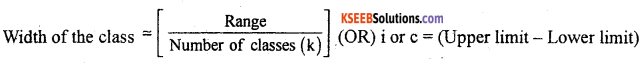Question 5.
What is a two-dimensional diagram?
In two dimensional diagram, both height and width (X and Y – axes) are considered.

Question 6.
Name the graph used to find the median.
Ogives/cumulative frequency curves.

Question 7.
Find the Harmonic mean of $$\frac { 1 }{ 4 }$$ and $$\frac { 1 }{ 6 }$$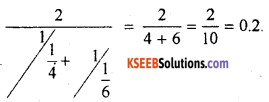Question 8.
For a data, Q2 = 30. Then what is the value of D5?
D5 = 30

Question 9.
Give one example for negative correlation.
Price and demand.

Question 10.
What is extrapolation?
Extrapolation is a procedure of estimating the unknown value of a dependent variable for a given value of an independent variable which is outside the limits or the range of the independent variable.

Question 11.
If S = {1, 2, 3, 4, 5, 6} and A {2, 4, 6} then find P (A1).
P(A1) = $$\frac { 3 }{ 6 }$$ = $$\frac { 1 }{ 2 }$$

Question 12.
Define probability distribution of a random variable.
A systematic presentation of the values taken by a variable with respective probabilities is called the probability distribution of a random variable.Section-B

II. Answer any ten of the following questions: (10 × 2 = 20)

Question 13.
Write any two functions of statistics.

1. It simplifies and condenses the size of the data.
2. If facilitates comparision.

Question 14.
Define census survey and sample survey.
Census method is the method of collection of data by complete enumeration of every unit of population.
Sample survey is the method of collection of data based on a part or portion of representative items from the population.

Question 15.
What is chronological classification? Give one example.
Chronological classification is the orderly arrangement of statistical data according to time.
eg: Classification of population of India from 1971 to 2011Question 16.
What are captions and stubs of a Table.

Question 17.
The mean age of a group of 50 students is 15 yrs and the mean age of another group of 70 students is 20 yrs. Find the mean age of all thel20 students together.
n1 = 50, X̄1 = 15; n2 = 70, X̄2 = 20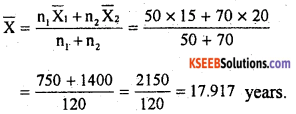Question 18.
The sum of the upper and lower quartiles is 90 and their difference is 30. Find the coefficient of quartile deviation. .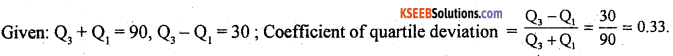Question 19.
Mention two uses of diagrams.

1. They give a birds eye view of the entire data at a glance.
2. They can be remembered for a longer period.

Question 20.
Name the different types of graphs.

• Histogram
• Frequency polygon
• Frequency curve
• Ogives.

Question 21.
Mention any two properties of regression coefficients.

1. Regression co-efficients are independent of the origin but dependent on the scale.
2. The geometric mean of the two regression co-efficients is numericilly equal to the co-efficient of correlation ie, γ = ± $$\sqrt{b x y \times b y x}$$

Question 22.
What is the difference between correlation coefficient and association of attributes.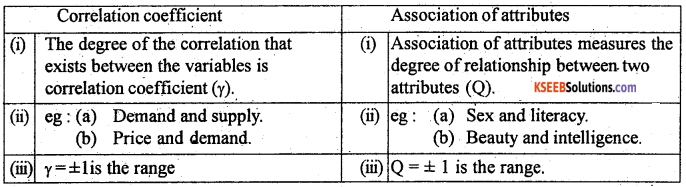Question 23.
Show that P (A) + P(A1) = 1.
Proof: we know that, if out of ‘n’ outcomes, ‘m’ outcomes are favorable to event A, then the remaining outcomes are favorable to event A1 – the complementary event of A.
By definition: P (A) = $$\frac { m }{ n }$$ and P (A1) = $$\frac { n-m }{ n }$$
P (A1) = $$\frac { n }{ n }$$ – $$\frac { m }{ n }$$ = 1 – $$\frac { m }{ n }$$ = 1 – P (A).
∴ P (A) + P (A1) = 1 GE.D.

Question 24.
If E(X) = 10 and SD (X) = 12, then find E(X2).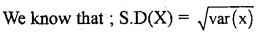Squaring both the sides,
[S.D (X)]2 = E(X2) – [E(X)]2
By substituting ; 122 = E (X2) – (10)2
∴ E(X2)= 144 – 100 = 44Section-C

III. Answer any eight of the following questions. (8 × 5 = 40)

Question 25.
What are the limitations of statistics?
The following are the limitations of statistics:

• It does not deal with qualitative data. It deals only with quantitative data.
• It does not deal with individual facts, but deals with aggregates of facts.
• Statistical results are not exact.
• It can be misused.
• Common people cannot handle statistics properly.

Question 26.
Mention the methods of collection of primary data. Explain any one method with their relative merit sand demerits. ‘
The important methods are:

• Direct personal interview method.
• Indirect investigation method.
• Local corresponding method.
• Questionnaire method.

Direct personal Interview method: In this method the investigator personally meets the informants. He puts a number of questions relating to the enquiry and collects necessary and desired information from the informants.
Merits:

• The information obtained is more accurate.
• Confusion, wrong interpretation of the questions by the respondants can be avoided.

Demerits :

• Time required is more.
• There is ar chance of personal influence on the outcome of the enquiry.

Question 27.
Prepare a blank table to show the distribution of employees in an office according to
(i) Sex : Men, Women
(ii) Ranks : Supervisors, assistants, clerks
(ii) Age groups (years) : 20-30, 30-50, 50-60 .
Blank table showing the distribution of employees of an office, according to age group, sex and rank.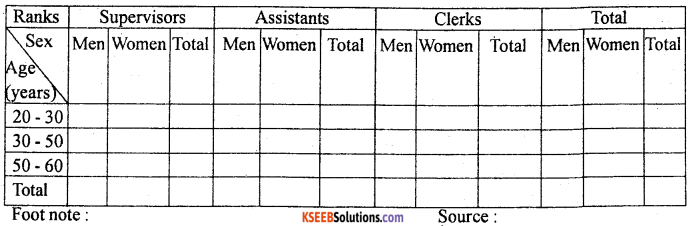Question 28.
Percentage break-up of the cost of construction of a house in Bengaluru is given below. Construct a pie diagram.

• Labour : 20%,
• Bricks : 12%,
• Cement : 20%
• Steel : 15%,
• Wood : 13%,
• Supervision : 15%,
• Other expenses : 5%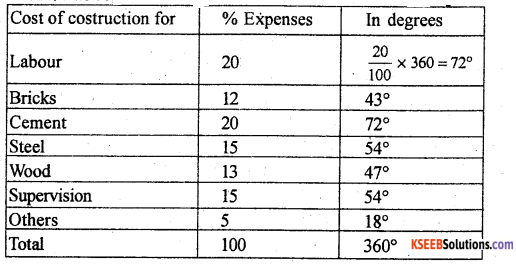Pie diagram showing the cost of constructing a house in BengaluruQuestion 29.
Compute mode for the following distribution regarding profit (in 000 Rs) of various firms.Let C.I and f be the profit (in 000 Rs) ad no.of firmsQuestion 30.
Following are the marks of 8 students in statistics and mathematics. Find Spearman’s rank correlation coefficient.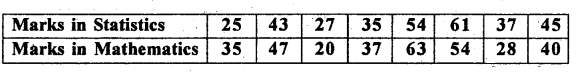Let x and y be the marks in statistic and mathematics and Rx and Ry be the respective ranks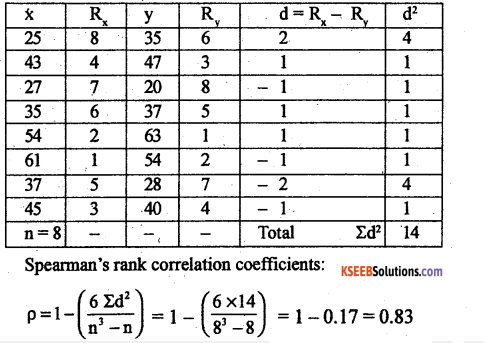There exists a high degree of positive correlation between marks in statistics and mathematics.

Question 31.
Calculate Karl Pearson’s coefficient of correlation between x and y.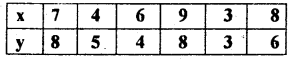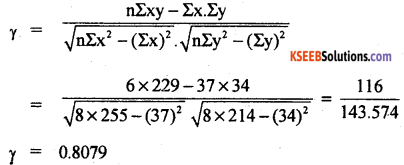There exists a high degree of positive correlation.Question 32.
Calculate Yule’s coefficient of association between marriage and failure of students for the following data pertaining to 525 students and comment.Let A – passed, α – failed and
B – married, β – unmarried
then, the 2 × 2 contingency table is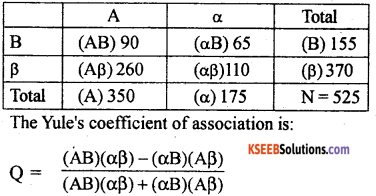There exists a low degree of negative association between marriage and failure.

Question 33.
Using suitable method of interpolation, estimate the sales (in lakhs) in 2004 from the following data.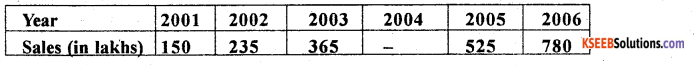Let x and y be the year and sales in lakhs.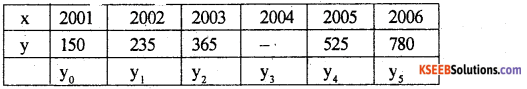There are five known values of y, so expand the Binominal expansion (y – 1)5 = 0
(y – 1)5 = y5 – 5y4 + 10y3 – 10y2 + 5y1 – y0 = 0,
780 – 5(525) + 10y3 – 10(365) + 5(235) – 150 = 0
780 – 2625 – 10y3 – 3650 + 1175- 150 = 0
y3 = 447 lakhs (sales)

Question 34.
State and prove addition theorem of probability for two non-mutually exclusive events.
Statement: Let A and B be any two events (Subsets of sample spaces) with respective probability P(A) and P(B). Then, the probability of occurence of atleast one of these events is:
P(A ∪ B) = P(A) + P(B) – P(A ∩ B)
where P(A ∩ B) is the simultaneous occurence of A and B.
Proof:
A random experiment results ‘n’ exhaustive outcomes out of which ‘m’ outcomes are favourable to event ‘A’, m2 outcomes are favourable to event ‘B’ and ‘p’ outcomes are common to both A and B.
Consider the venn diagram :The event of occurrence of atleast one out of A and B is (A ∪ B) has (m1 + m2 – p) favourable outcomes.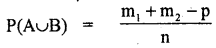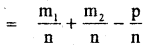Substituting the values from (1)
P(A ∪ B) = P(A) + P(B) – P(A ∩ B)
Q.E.D.Question 35.
For a University cricket team, 2 players are to be selected from a certain College among 5 batsmen, 3 bowlers and 2 wicket keepers. Find the probability of selecting
(i) a batsman and a wicket keeper
(ii) no wicket keeper.
n = Total number outcomes to select 2 players from (5 batsmen + 3 bowlers + 2 wicket keepers) 10 players = 10C2 = 45 ways

(i) m = One batsman out of 5 batsmen and one wicket keeper out of can be 2 selected in (5C1 × 2C1) = 5 × 2 = 10 outcomes.
P (Selecting one batsman and one wicket keeper)
$$=\frac{\mathrm{m}}{\mathrm{n}}=\frac{10}{45}=\frac{2}{9}$$

(ii) m = no wicket keeper which means both the players are either batsmen or bowlers.
= 8C2 = 28
P (Selecting two players but no wicket keeper) = $$\frac { m }{ n }$$ = $$\frac { 28 }{ 45 }$$

Question 36.
For the following probability distribution, find mean and variance.Mean = E(x) = ΣX.(PX)
= 1 × $$\frac { 2 }{ 3 }$$ + 5 × $$\frac { 1 }{ 3 }$$
E(x) = $$\frac { 7 }{ 3 }$$ = 2.33
Var (x) – E(x2) – [E(x)2]
E(x2) = Σx2.P(x)
$$=1^{2} \times \frac{2}{3}+5^{2} \times \frac{1}{3}=\frac{2}{3}+\frac{25}{3}=\frac{27}{3}=9$$
∴ V(x) = 9 – (2.33)2
= 3.57

section-D

IV. Answer any two of the following questions: (2 × 10 = 20)

Question 37.
The number of runs scored by two batsmen A and B in different innings are as follows.(i) Who is better run scorer?
(ii) Who is more consistant?
Let x1 and x2 be the runs of batsmen A and B.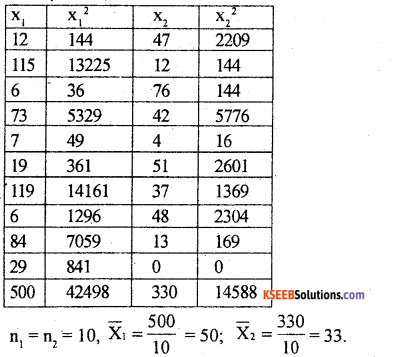(i) Here X̄1(batsman A) > X̄2 (batsman B)
So batsman A is the better run scorer.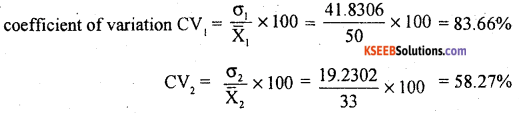Here CV2 (batsman B) < CV1, (batsman A)
∴ Batsman B is more consistant.Question 38.
Calculate Karl – Pearson’s coefficient of skewness from the following data.The frequency distribution is bi-modal, so using Karl-Pearson’s coefficient of skewness for ill-defined data: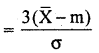Let C.I and f be the Age and no.of persons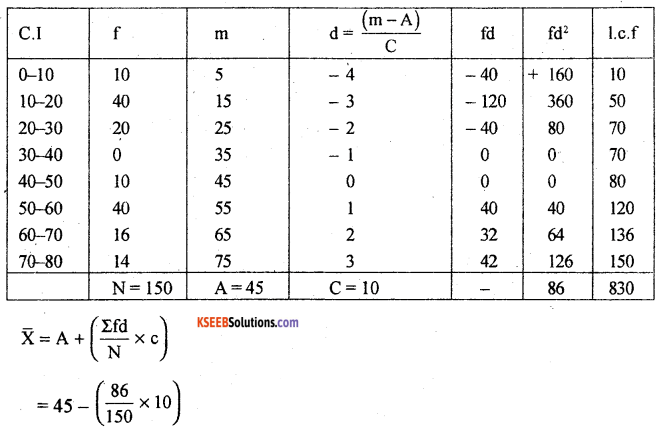The distribution is negatively skewed.Question 39.
From the following data regarding the amount of rainfall (x) and the production of rice (y).
1. Find the most likely production corresponding to a rainfall of 50 cms.
2. Find the most likely rainfall corresponding to the production of 45 quintals.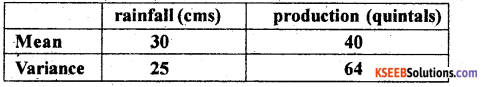Coefficient of correlation = 0.6
Let x and y be the rainfall and production
given: x̄ = 30; ȳ = 40, = σ2x 25 ∴ σx = 5
σ2y =64 ∴ σ2y =8; γ= 0.6

1. To find y when x = 50; we need the regression equation of y on x:
(y – ȳ) = byx (x – x̄)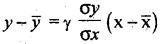y – 40 = 0.6 × $$\frac { 8 }{ 5 }$$ (50 – 30) = 0.96 × 20
y = 19.2 + 40 = 59.2 quintals.

2. To find x when y = 45, we need the regression equation of x on y: (x – x̄) = b × y (y – ȳ)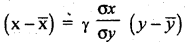X – 30 = 0.6 × $$\frac { 5 }{ 8 }$$ (45 – 40) = 0.375 × 5
X = 1.875+ 30 = 31.875 cms rainfall.

Question 40.
From the following joint probability distribution of x and y, find the correlation coefficient r.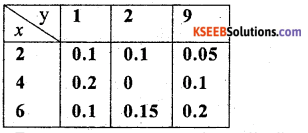From the bi -variate probability distribution, the marginal distribution of x is: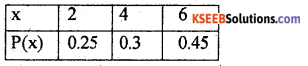E(x) = Σx. P(x) = 2 × 0.25 + 4 × 0.3 + 6 × 0.45
E(x) = 4.4
E(x2) = 22 × 0.25 + 42 × 0.3 + 62 × 0.45 = 22
Var (x) = E(x2) – [E(x)]2 = 22 – (4.4)2 = 2.64.
The marginal proabability distribution of y: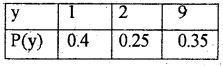E(y) = Σy. P(y) = 1 × 0.4 + 2 × 0.25 + 9 × 0.35
E(y) = 4.05
E(y2) = 12 × 0.4 + 22 × 0.25 + 92 × 0.35
= 29.75
Var(y) = E(y2) – [E(y)]2
= 29.75 – (4.05)2 = 13.3475 ,= (2 × 1 × 0.1) + (4 × 1 × 0.2) + (6 × 0.1 × 1) + (2 × 2 × 0.1) + (4 × 2 × 0) + (6 × 2 × 0.15) + (2 × 9 × 0.05) + (4 × 9 × 0.1) + (6 × 9 × 0.2)
= 0.2 + 0.8 + 0.6 + 0.4 + 0 + 1.8 + 0.9 + 3.6 + 10.8
E(x + y) = 19.1
Con (x,y)= E(x,y) – E(x) . E(y)
= 19.1 – 4.4 × 4.05
= 1.28.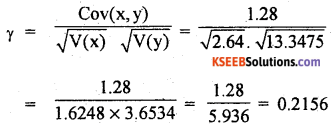Section-E

V. Answer any two of the following questions (2 × 5 = 10)

Question 41.
Given below are the daily wages in rupees of 36 workers in a factory manufacturing plastic products. Form a frequency distribution by exclusive Cl, taking lowest class interval as (90 – 140).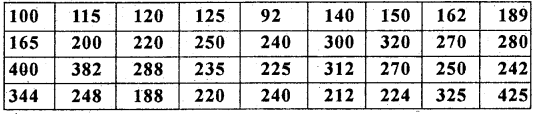Frequency table of daily wages of factory workers:Question 42.
Draw a histogram for the following data and hence find mode.Histogram of daily wages of workers, (in ₹s)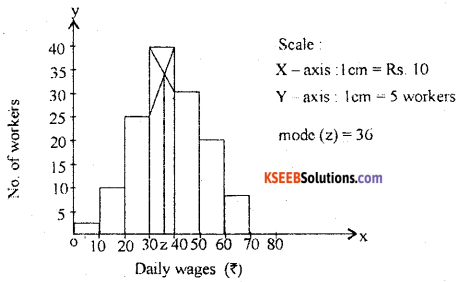Question 43.
Calculate D3 and P10 from the following data.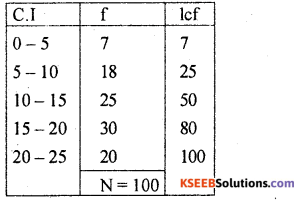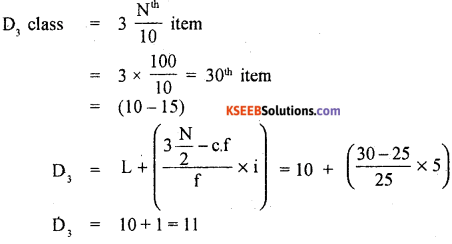Question 44.
The probabilities of a husband and his wife surviving for 20 more years are 0.8 and 0.9 respectively. Find the probability that after 20 years,
(i) both of them are alive
(ii) atleast one of them is alive